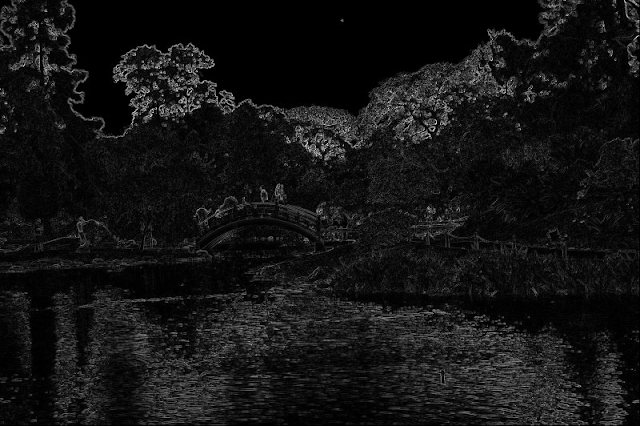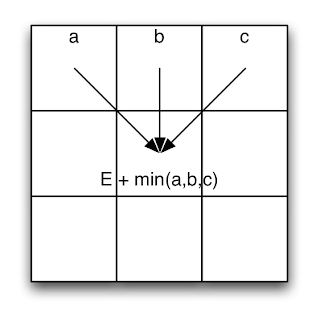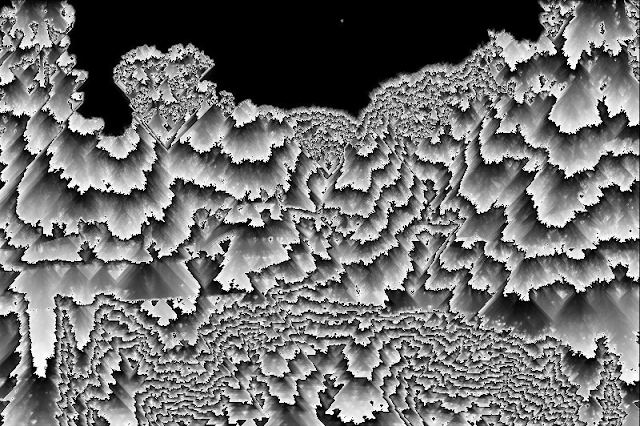Today I’m going to discuss a technique called Seam Carving, originally presented in Siggraph 2007. This algorithm at it’s core it’s fairly simple but produces impressive results.

We will start from this image:And take 200 pixels from its width, and turn it into this one:Note that the image wasn’t just resized, but most of the detail is still there. The size reduction is rather aggressive so there are some artifacts. But the results are quite good.

This algorithm works by repeatedly finding vertical seams of pixels and removing them. It chooses which one to remove by finding the seam with the minimal amount of energy.

The whole algorithm revolves around an energy function. In this case, I’m using a function suggested in the original paper which is based on the luminance of the image. What we do is compute the vertical and horizontal derivatives of the image, take the absolute value of each, and add both. The derivative is approximated by a simple subtraction.

The following code computes the energy of the image. The intensities image is basically the grayscale version of the image, normalized between 0 and 1.

private static FloatImage computeEnergy(FloatImage intensities) {
int w = intensities.getWidth(), h = intensities.getHeight();
final FloatImage energy = FloatImage.createSameSize(intensities);
for(int x = 0; x < w-1; x++) {
for(int y = 0; y < h-1; y++) {
//I'm aproximating the derivatives by subtraction
float e = abs(intensities.get(x,y)-intensities.get(x+1,y))
+ abs(intensities.get(x,y)-intensities.get(x,y+1));
energy.set(x,y, e);
}
}
return energy;
}


After applying this function to our image, we get the following:You can observe that the edges are highlighted (i.e. have more energy). That is caused by our choice of an energy function. Since we’re taking the derivatives and adding its absolute value, abrupt changes in luminance are highlighted (i.e. edges). The next step is where things start to get interesting. To find the minimal energy seam, we build an image with the accumulated minimal energy. We do so by computing an image where the value of each pixel is the value of the minimum of the three above it, plus the energy of that pixel:We do so with the following code:

final FloatImage energy = computeEnergy(intensities);

final FloatImage minima = FloatImage.createSameSize(energy);
//First row is equal to the energy
for(int x = 0; x < w; x++) {
minima.set(x,0, energy.get(x,0));
}

//I assume that the rightmost pixel column in the energy image is garbage
for(int y = 1; y < h; y++) {
minima.set(0,y, energy.get(0,y) + min(minima.get(0, y - 1),
minima.get(1, y - 1)));

for(int x = 1; x < w-2; x++) {
final float sum = energy.get(x,y) + min(min(minima.get(x - 1, y - 1),
minima.get(x, y - 1)),minima.get(x + 1, y - 1));
minima.set(x,y, sum);
}
minima.set(w-2,y, energy.get(w-2,y) + min(minima.get(w-2, y - 1),
minima.get(w-3, y - 1)));
}


Once we do this, the last row contains the sum of all the potential minimal seams.With this, we search the last row for the one with the minimum total value:

//We find the minimum seam
float minSum = Float.MAX_VALUE;
int seamTip = -1;
for(int x = 1; x < w-1; x++) {
final float v = minima.get(x, h-1);
if(v < minSum) {
minSum=v;
seamTip=x;
}
}


And backtrace the seam:

//Backtrace the seam
final int[] seam = new int[h];
seam[h-1]=seamTip;
for(int x = seamTip, y = h-1; y > 0; y--) {
float left = x>0?minima.get(x-1, y-1):Float.MAX_VALUE;
float up = minima.get(x, y-1);
float right = x+1<w?minima.get(x+1, y-1):Float.MAX_VALUE;
if(left < up && left < right) x=x-1;
else if(right < up && right < left) x= x+1;
seam[y-1]=x;
}


Having the minimum energy seam, all is left to do is remove it.

If we repeat this process several times, removing one seam at a time, we end up with a smaller image. Check the following video to see this algorithm in action:

If you want to reduce an image vertically, you have to find horizontal seams. If you want to do it vertically and horizontally you have to find which seam has the least energy (either the vertical or the horizontal one) and remove that one. This implementation is quick & dirty and very simplistic. Many optimization can be done to make it work faster. It is also quite incomplete. By priming the energy image, you can influence the algorithm to avoid distorting certain objects in the image or to particularly pick one. It is also possible to use it to enlarge an image (although I haven’t implemented it), and by a combination of both methods one can selectively remove objects from an image. The full source code for this demo follows. Have fun!

import javax.imageio.ImageIO;
import java.io.File;
import java.io.IOException;
import java.awt.image.BufferedImage;
import java.awt.*;
import static java.lang.Math.abs;
import static java.lang.Math.min;

public class SeamCarving
{
public static void main(String[] args) throws IOException {
final BufferedImage input = ImageIO.read(new File(args));

final BufferedImage[] toPaint = new BufferedImage[]{input};
final Frame frame = new Frame("Seams") {

@Override
public void update(Graphics g) {
final BufferedImage im = toPaint;
if (im != null) {
g.clearRect(0,0,getWidth(), getHeight());
g.drawImage(im,0,0,this);
}
}
};
frame.setSize(input.getWidth(), input.getHeight());
frame.setVisible(true);

BufferedImage out = input;
for(int i = 0; i < 200; i++) {
out = deleteVerticalSeam(out);
toPaint=out;
frame.repaint();
}
}

private static BufferedImage deleteVerticalSeam(BufferedImage input) {
return deleteVerticalSeam(input, findVerticalSeam(input));
}

private static BufferedImage deleteVerticalSeam(final BufferedImage input, final int[] seam) {
int w = input.getWidth(), h = input.getHeight();
final BufferedImage out = new BufferedImage(w-1,h, BufferedImage.TYPE_INT_ARGB);

for(int y = 0; y < h; y++) {
for(int x = 0; x < seam[y]; x++) {
out.setRGB(x,y,input.getRGB(x, y));
}
for(int x = seam[y]+1; x < w; x++) {
out.setRGB(x-1,y,input.getRGB(x, y));
}
}
return out;
}

private static int[] findVerticalSeam(BufferedImage input) {
final int w = input.getWidth(), h = input.getHeight();
final FloatImage intensities = FloatImage.fromBufferedImage(input);
final FloatImage energy = computeEnergy(intensities);

final FloatImage minima = FloatImage.createSameSize(energy);
//First row is equal to the energy
for(int x = 0; x < w; x++) {
minima.set(x,0, energy.get(x,0));
}

//I assume that the rightmost pixel column in the energy image is garbage
for(int y = 1; y < h; y++) {
minima.set(0,y, energy.get(0,y) + min(minima.get(0, y - 1),
minima.get(1, y - 1)));

for(int x = 1; x < w-2; x++) {
final float sum = energy.get(x,y) + min(min(minima.get(x - 1, y - 1),
minima.get(x, y - 1)),minima.get(x + 1, y - 1));
minima.set(x,y, sum);
}
minima.set(w-2,y, energy.get(w-2,y) + min(minima.get(w-2, y - 1),minima.get(w-3, y - 1)));
}

//We find the minimum seam
float minSum = Float.MAX_VALUE;
int seamTip = -1;
for(int x = 1; x < w-1; x++) {
final float v = minima.get(x, h-1);
if(v < minSum) {
minSum=v;
seamTip=x;
}
}

//Backtrace the seam
final int[] seam = new int[h];
seam[h-1]=seamTip;
for(int x = seamTip, y = h-1; y > 0; y--) {
float left = x>0?minima.get(x-1, y-1):Float.MAX_VALUE;
float up = minima.get(x, y-1);
float right = x+1<w?minima.get(x+1, y-1):Float.MAX_VALUE;
if(left < up && left < right) x=x-1;
else if(right < up && right < left) x= x+1;
seam[y-1]=x;
}

return seam;
}

private static FloatImage computeEnergy(FloatImage intensities) {
int w = intensities.getWidth(), h = intensities.getHeight();
final FloatImage energy = FloatImage.createSameSize(intensities);
for(int x = 0; x < w-1; x++) {
for(int y = 0; y < h-1; y++) {
//I'm approximating the derivatives by subtraction
float e = abs(intensities.get(x,y)-intensities.get(x+1,y))
+ abs(intensities.get(x,y)-intensities.get(x,y+1));
energy.set(x,y, e);
}
}
return energy;
}
}

import java.awt.image.BufferedImage;

public final class FloatImage {
private final int width;
private final int height;
private final float[] data;

public FloatImage(int width, int height) {
this.width = width;
this.height = height;
this.data = new float[width*height];
}

public int getWidth() {
return width;
}

public int getHeight() {
return height;
}

public float get(final int x, final int y) {
if(x < 0 || x >= width) throw new IllegalArgumentException("x: " + x);
if(y < 0 || y >= height) throw new IllegalArgumentException("y: " + y);
return data[x+y*width];
}

public void set(final int x, final int y, float value) {
if(x < 0 || x >= width) throw new IllegalArgumentException("x: " + x);
if(y < 0 || y >= height) throw new IllegalArgumentException("y: " + y);
data[x+y*width] = value;
}

public static FloatImage createSameSize(final BufferedImage sample) {
return new FloatImage(sample.getWidth(), sample.getHeight());
}

public static FloatImage createSameSize(final FloatImage sample) {
return new FloatImage(sample.getWidth(), sample.getHeight());
}

public static FloatImage fromBufferedImage(final BufferedImage src) {
final int width = src.getWidth();
final int height = src.getHeight();
final FloatImage result = new FloatImage(width, height);
for(int x = 0; x < width; x++) {
for(int y = 0; y < height; y++) {
final int argb = src.getRGB(x, y);
int r = (argb >>> 16) & 0xFF;
int g = (argb >>> 8) & 0xFF;
int b = argb & 0xFF;
result.set(x,y, (r*0.3f+g*0.59f+b*0.11f)/255);
}
}
return result;
}
public BufferedImage toBufferedImage(float scale) {
final BufferedImage result = new BufferedImage(width, height, BufferedImage.TYPE_INT_ARGB);
for(int x = 0; x < width; x++) {
for(int y = 0; y < height; y++) {
final int intensity = ((int) (get(x, y) * scale)) & 0xFF;
result.setRGB(x,y,0xFF000000 | intensity | intensity << 8 | intensity << 16);
}
}
return result;
}
}